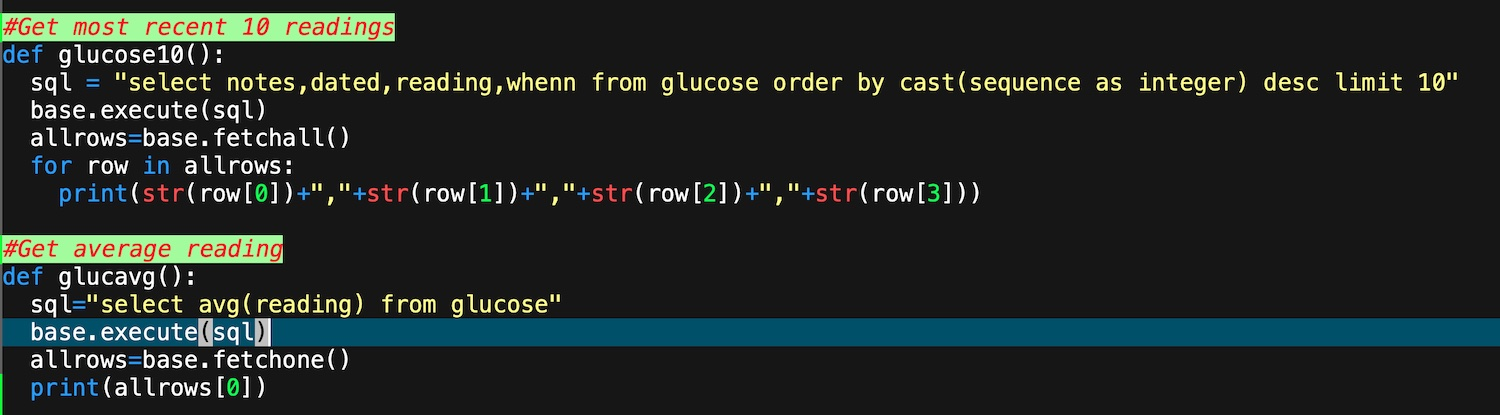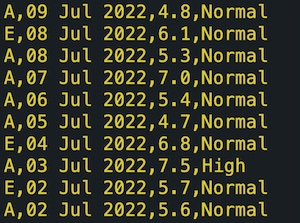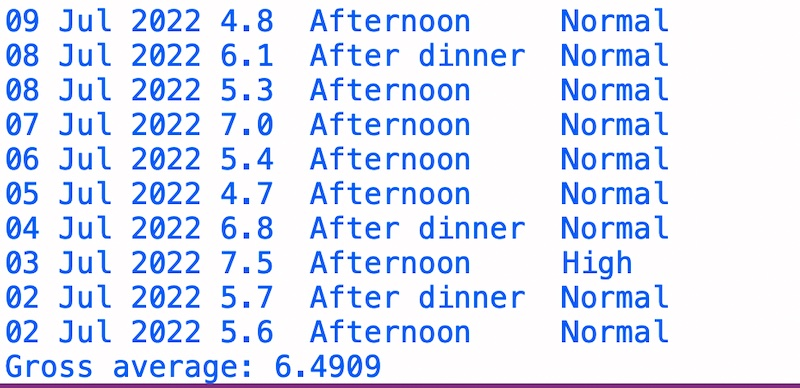An Application Programming Interface in Python
My main programming language for general use on the Mac is XOJO. In general, my XOJO applications use a MySQL database resident on the web server, (see my page about that. The database is fast and works well for these web pages, but I find that a local XOJO application can be slow to retrieve data from the database.

On the other hand, I found that a Python approach to the database was fast and efficient. So I wondered if I could combine the efficiency and GUI-capable approach of XOJO with an underlying Python database API. I experimented.

Like, I am sure, most programming languages, XOJO has the ability to run an application in a shell and to retrieve that application's result, if any. I experimented, and wrote a small Python application and experimented with it using a XOJO test application. Yes, of course it worked. How could I leverage this into my main XOJO apps?

As I continued to experiment I decided to further my experiments using my Glucose data. I have an extensive XOJO appliation which both records glucose readings as well as analyses them. See my diabetes page which explains how glucose readings are used.

The Python program starts:

``` import mysql.connector import sys import functions #Personal set of manipulation functions if len(sys.argv)==1:   print("Bad call")   #First argv will always be program name - we want at least 2   sys.exit() what=sys.argv #This will be the name of the function to execute #Connect: db=mysql.connector.connect(user='1234',password='1234',host='1234',database='1234') #Cursor: base=db.cursor() ```

Then right at the bottom of the Python code is

``` eval(what+()) #Execute the function in what db.close ```

Here are a couple of my Python functions for glucose:Here, in a terminal window, is the output from function glucose10():Function glucavg() simply returns a single floating point (in a string) value: 6.490937996820354, to be rounded in the XOJO side.

So we know that Python gives us what we want. Now, how to get the Python output into XOJO? I have a function called api() in XOJO (called a Method) which is:

``` var sh as new shell sh.execute("/usr/local/bin/python3 /Volumes/Molybdenum/Python/api.py "+param) return sh.result ```
Here, param is the value passed to the method.

In the mainline code in XOJO we have:

``` var a() as string var b() as string var w as string var x as integer var readwhen as string result.text=api("glucose10") a=result.text.split(chr(10)) for x=0 to ubound(a)-1   b=a(x).split(",")   if b(0)="E" then readwhen="After dinner" else readwhen="Afternoon "   w=b(1)+" "+b(2)+" "+readwhen+" "+b(3)   lbox.addrow(w) next ```

Explanation:
result is a textarea; it receives the Python output
a() is an array; it receives the split by line break of the Python output, which is seen above
Then, each of a() is further split by comma into b(), another array